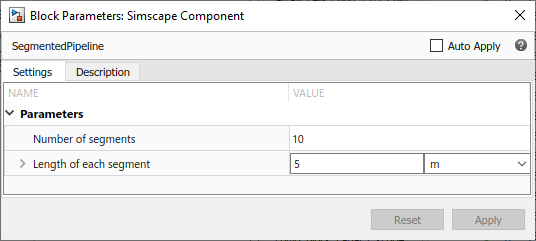## Segmented Pipeline Using Component Array

This example shows how you can model a segmented pipeline using a component array. This segmented pipeline model is a composite component that consists of `N` identical segments connected in series. Individual pipe segments are represented by the Pipe (IL) blocks from the Foundation library. `N` is a parameter that the block user can modify.

```component SegmentedPipeline parameters N = 10; % Number of segments segm_length = { 5, 'm' }; % Length of each segment end % Ports at the two ends of the pipeline nodes A = foundation.isothermal_liquid.isothermal_liquid; % A:left B = foundation.isothermal_liquid.isothermal_liquid; % B:right end % Declare array of N components for i=1:N components (ExternalAccess=none) pipe(i) = foundation.isothermal_liquid.elements.pipe(length = segm_length); end end % Connect all segments in series for i=1:(N-1) connections connect(pipe(i).B, pipe(i+1).A); end end % Connect two ends of pipeline to first and last segment, respectively connections connect(A, pipe(1).A); connect(B, pipe(N).B); end end ```

In this example, for the sake of simplicity, the `SegmentedPipeline` component has only two modifiable parameters: `N` (Number of segments) and `segm_length` (Length of each segment). However, you can make other parameters of the underlying Pipe (IL) block accessible from the top-level composite component block dialog, as described in Parameterizing Composite Components. Parameter `N`, which defines the array size and is going to be used as the upper limit for the `for`-loop iterator, is declared as a unitless integer.

Use a `for`-loop to declare an array of `N` member components:

``` for i=1:N components (ExternalAccess=none) pipe(i) = foundation.isothermal_liquid.elements.pipe(length = segm_length); end end ```

In this example, all the pipe segments have the same length. For an example of array component members having different parameter values, see Case Study — Battery Pack with Fault Using Arrays.

Use another `for`-loop to connect all segments in series, by connecting node `B` of each pipe segment (except the last one) to node `A` of the next segment:

``` for i=1:(N-1) connections connect(pipe(i).B, pipe(i+1).A); end end ```

Finally, connect the internal chain of segments to the two ends of pipeline, by connecting node `A` of the composite component to node `A` of the first segment and connecting node `B` of the composite component to node `B` of the last segment:

``` connections connect(A, pipe(1).A); connect(B, pipe(N).B); end end ```

The resulting block has two isothermal liquid ports, A and B, and two modifiable parameters: Number of segments and Length of each segment.